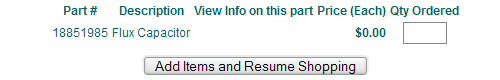# Quick Statistics Question

Scritch scratch scritch scratch scritch scratch scritch scratch scritch scratch scritch scratchscritch scratch scritch scratch scritch scratch scritch scratch scritch scratch scritch scratchRegistered User regular
edited March 2009
Okay, so I have a Univariate Statistics test tomorrow morning. I'm going through the practice problems that the professor posted online, and I'm a little confused about one of the problems.

The problem is:

6. Orthogonal Comparisons
Compare Group D to the other three groups.
Group A raw data: 5 5 4 3 (total=17)
Group B raw data: 4 2 4 4 (total=14)
Group C raw data: 9 9 8 7 (total=33)
Group D raw data: 9 7 7 7 (total=30)
The SSbetween for this problem = 66.25 and the SSwithin = 11.50

When I calculate this out, I get the sums of squares to be 28.166... But on the answer key to the practice problems, the sums of squares is listed as 14.0833..., which is exactly half of what I got. This happens even when I punch the exact steps that the answer key lists into my calculator, which leads me to believe that there's some sort of step that's supposed to be obvious or implicit that I'm overlooking. What I need to know is, after I do:

(ψ^2)/(Σa)

Do I just divide the answer by 2 every time, or is there something I need to do to figure out how much I need to divide by? All of the practice problems use the same set of data or I could figure this out on my own.

What makes it even more confusing is that a later problem asks to compare Group A to Group B, and on that one I calculate the same sums of squares as the answer key without needing to do any dividing.

Help would be appreciated! Hopefully what I typed was coherent enough for someone to understand!http://www.audioentropy.com/
Speed Racer on

## Posts

• edited March 2009
(ψ^2)/(Σa)

This equation is incorrect, which is why you are getting the wrong answer. You're comparing three groups against one, which means that you need to use coefficients to weight the groups accordingly. The correct equation to use:

SScomp = n(ψ)^2 / Σc^2

Where n is the size of each group, ψ is the sum of the weighted means by the coefficients, and Σc^2 is the sum of the squared coefficients.

First, the coefficients. You are comparing A, B, and C to D. I'm representing this below:

A B C | D

You need to weight the groups so that they are balanced. The sum of the weights on both sides of | needs to be zero. For your example, you could do this:

(1/3)A (1/3)B (1/3)C | (-1)D

Make sense?

So,
n = 4
Σc^2 = (1/3)^2 + (1/3)^2 + (1/3)^2 + (-1)^2 = 1.33
ψ = (1/3)4.25 + (1/3)3.5 + (1/3)8.25 + (-1)7.5 = -2.17

Putting everything into the equation:

SScomp = 4(-2.17)^2 / 1.33 = 14.16 (<<<I was really lazy with rounding, but that's right).

littwentythree on• edited March 2009
Holy shit. I too have a stats test tomorrow morning

Nothing like that though...

basic probabilitymooshoepork on
Flickr!• Scritch scratch scritch scratch scritch scratch scritch scratch scritch scratch scritch scratch scritch scratch scritch scratch scritch scratch scritch scratch scritch scratch scritch scratchRegistered User regular
edited March 2009
(ψ^2)/(Σa)

This equation is incorrect, which is why you are getting the wrong answer. You're comparing three groups against one, which means that you need to use coefficients to weight the groups accordingly. The correct equation to use:

SScomp = n(ψ)^2 / Σc^2

Where n is the size of each group, ψ is the sum of the weighted means by the coefficients, and Σc^2 is the sum of the squared coefficients.

First, the coefficients. You are comparing A, B, and C to D. I'm representing this below:

A B C | D

You need to weight the groups so that they are balanced. The sum of the weights on both sides of | needs to be zero. For your example, you could do this:

(1/3)A (1/3)B (1/3)C | (-1)D

Make sense?

So,
n = 4
Σc^2 = (1/3)^2 + (1/3)^2 + (1/3)^2 + (-1)^2 = 1.33
ψ = (1/3)4.25 + (1/3)3.5 + (1/3)8.25 + (-1)7.5 = -2.17

Putting everything into the equation:

SScomp = 4(-2.17)^2 / 1.33 = 14.16 (<<<I was really lazy with rounding, but that's right).

Yup, there we go! Unfortunately, the practice test didn't really reflect the real one. It was more "Design a scenario in which you'd use two-way ANOVA" and less "Here's some data, do some statistics," so I kind of bombed it!

Thanks for the help anyway, though!

Speed Racer onhttp://www.audioentropy.com/
• edited March 2009
Bummer :\

littwentythree on